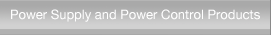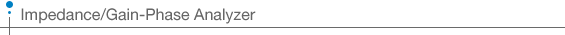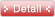home > Products > Measurement Instruments >Impedance/Gain-Phase AnalyzerSignal GeneratorLCR MeterImpedance/Gain-Phase AnalyzerAC Voltmeter/Noise MeterLock-in Amplifier/PreamplifierFilterMeasurement SystemFrequency Response AnalyzerImpedance/Gain-Phase Analyzer ZGA5905A/D converted input signals undergo discrete Fourier transform (DFT) to calculate complex impedance values and obtain parameters and characteristics specific to the DUT, such as its capacitance, inductance and quality factor. Original NF algorithms are also applied to allow equivalent circuits made up of R, L and C along with the constants for those circuits to be estimated from the complex impedance spectrum obtained by sweeping the frequencies. In servo analysis, data such as the loop cycle gain and closed-loop gain are used to obtain transfer functions (circuit model) and run simulations of the loop cycle gain and closed-loop gain.
 Model Description ZGA5920 Impedance/gain-phase analyzerFRA5097 Frequency Response Analyzer